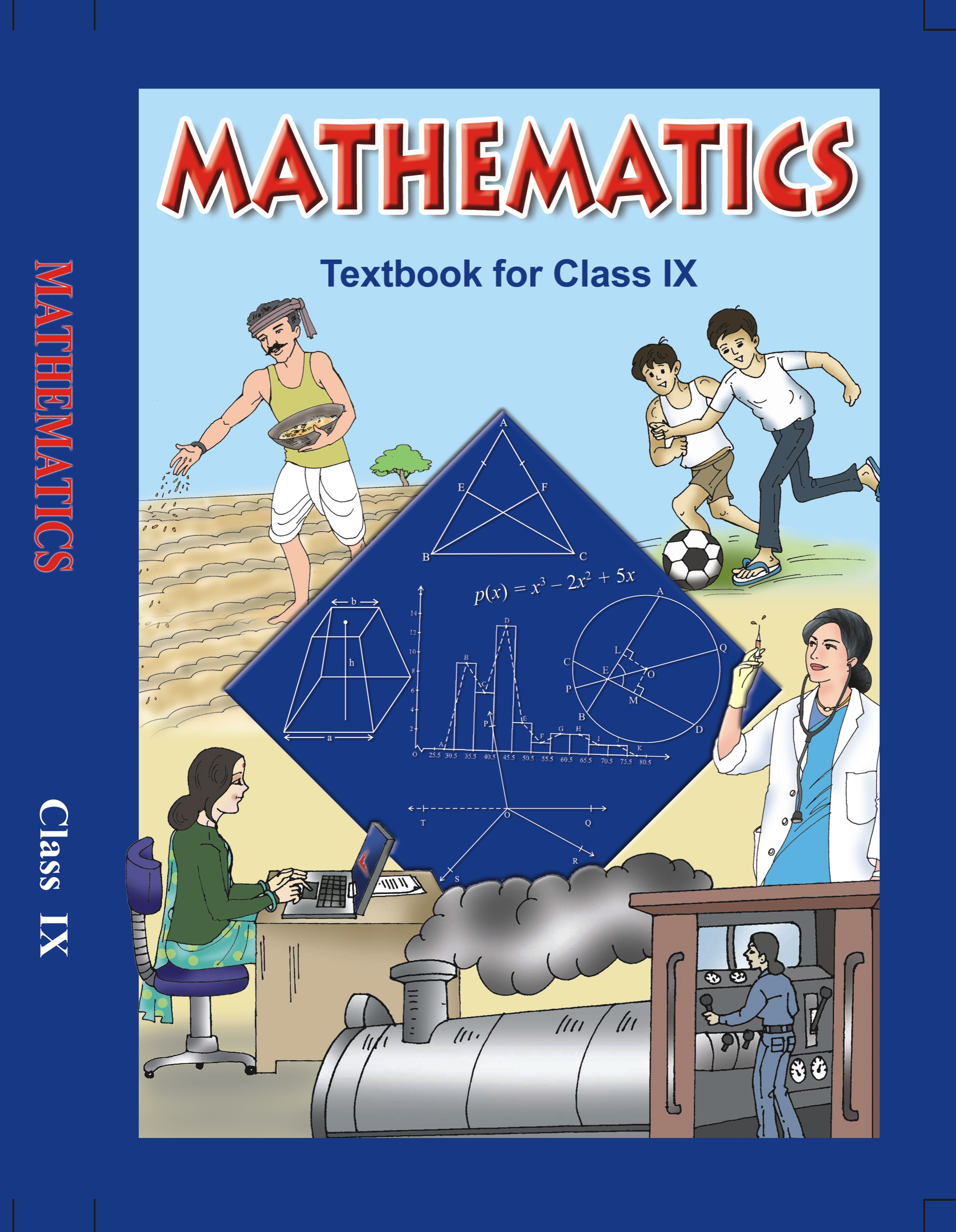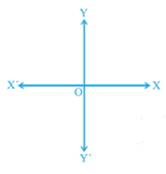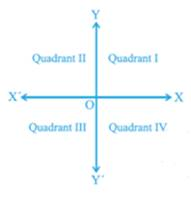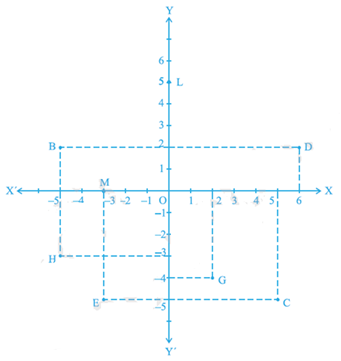# NCERT Solutions for Class 9 Maths Exercise 3.2## myCBSEguide App

CBSE, NCERT, JEE Main, NEET-UG, NDA, Exam Papers, Question Bank, NCERT Solutions, Exemplars, Revision Notes, Free Videos, MCQ Tests & more.

NCERT solutions for Class 9 Maths Coordinate Geometry## NCERT Solutions for Class 9 Math Coordinate Geometry

###### 1. Write the answer of each of the following questions:

(i) What is the name of horizontal and the vertical lines drawn to determine the position of any point in the Cartesian plane?

(ii) What is the name of each part of the plane formed by these two lines?

(iii) Write the name of the point where these two lines intersect.

Ans. (i) The horizontal line that is drawn to determine the position of any point in the Cartesian plane is called as x-axis.

The vertical line that is drawn to determine the position of any point in the Cartesian plane is called as y-axis.(ii) The name of each part of the plane that is formed by x-axis and y-axis is called as quadrant.(iii) The point, where the x-axis and the y-axis intersect is called as origin.

NCERT Solutions for Class 9 Maths Exercise 3.2

###### 2. See Fig.3.14, and write the following:

(i) The coordinates of B.

(ii)The coordinates of C.

(iii)The point identified by the coordinates (–3, –5).

(iv) The point identified by the coordinates (2, – 4).

(v) The abscissa of the point D.

(vi) The ordinate of the point H.

(vii) The coordinates of the point L.

(viii) The coordinates of the point M.Ans. We need to consider the given below figure to answer the following questions.

(i) The coordinates of point B in the above figure is the distance of point B from x-axis and y-axis. Therefore, we can conclude that the coordinates of point B are (―5, 2).

(ii) The coordinates of point C in the above figure is the distance of point C from x-axis and y-axis. Therefore, we can conclude that the coordinates of point C are (5, ―5).

(iii) The point that represents the coordinates (―3, ―5) is E.

(iv) The point that represents the coordinates (2, ―4) is G.

(v) The abscissa of point D in the above figure is the distance of point D from the y-axis. Therefore, we can conclude that the abscissa of point D is 6.

(vi) The ordinate of point H in the above figure is the distance of point H from the x-axis. Therefore, we can conclude that the abscissa of point H is ―3.

(vii) The coordinates of point L in the above figure is the distance of point L from x-axis and y-axis. Therefore, we can conclude that the coordinates of point L are (0, 5).

(viii) The coordinates of point M in the above figure is the distance of point M from x-axis and y-axis. Therefore, we can conclude that the coordinates of point M are (―3, 0).

## NCERT Solutions for Class 9 Maths Exercise 3.2

NCERT Solutions for Class 9 Maths Exercise 3.2 PDF (Download) Free from myCBSEguide app and myCBSEguide website. Ncert solution class 9 Maths includes text book solutions from Mathematics Book. NCERT Solutions for CBSE Class 9 Maths have total 15 chapters. 9 Maths NCERT Solutions in PDF for free Download on our website. Ncert Maths class 9 solutions PDF and Maths ncert class 9 PDF solutions with latest modifications and as per the latest CBSE syllabus are only available in myCBSEguide.

## CBSE app for Class 9

To download NCERT Solutions for Class 9 Maths, Computer Science, Home Science,Hindi ,English, Social Science do check myCBSEguide app or website. myCBSEguide provides sample papers with solution, test papers for chapter-wise practice, NCERT solutions, NCERT Exemplar solutions, quick revision notes for ready reference, CBSE guess papers and CBSE important question papers. Sample Paper all are made available through the best app for CBSE students and myCBSEguide website.Test Generator

Create question papers online with solution using our databank of 5,00,000+ questions and download as PDF with your own name & logo in minutes.